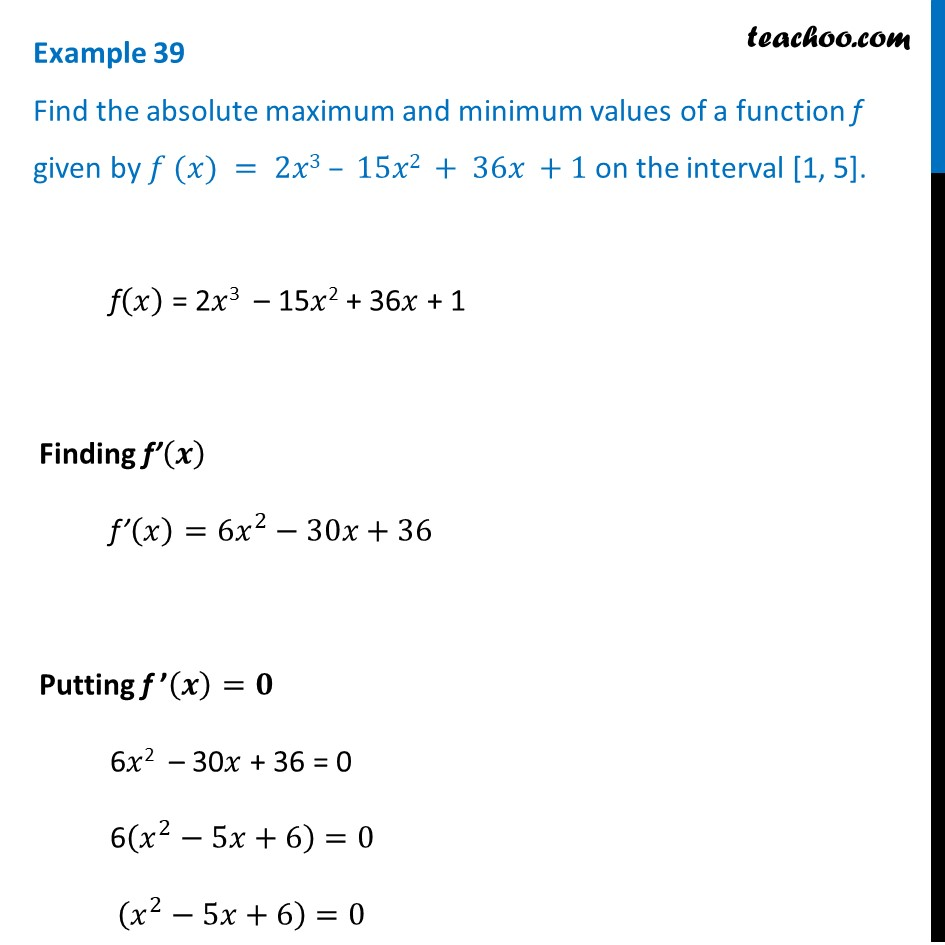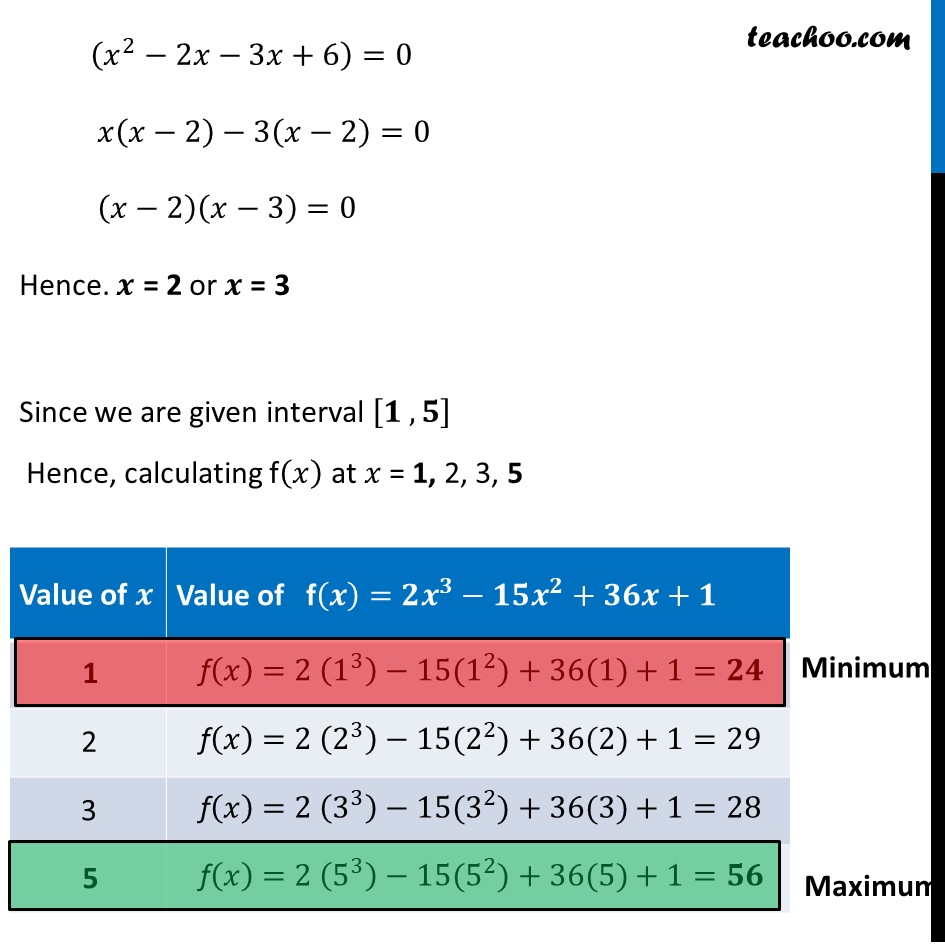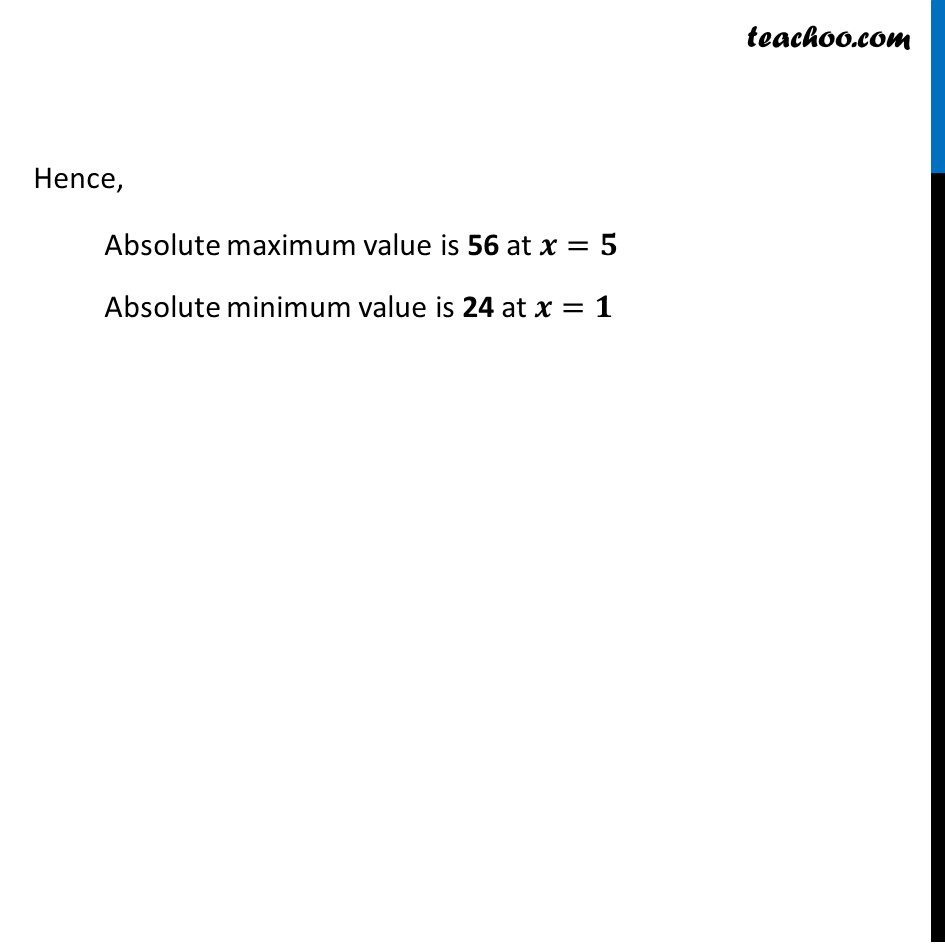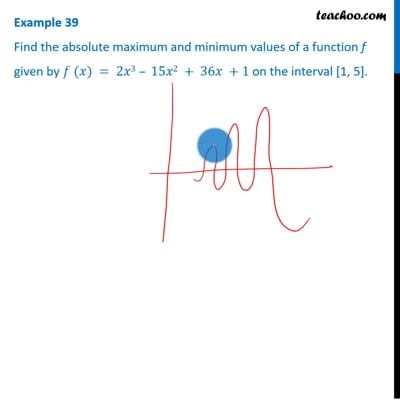Examples

Chapter 6 Class 12 Application of Derivatives
Serial order wiseThis video is only available for Teachoo black users

Learn in your speed, with individual attention - Teachoo Maths 1-on-1 Class

### Transcript

Example 27 Find the absolute maximum and minimum values of a function f given by 𝑓 (𝑥) = 2𝑥3 – 15𝑥2 + 36𝑥 +1 on the interval [1, 5]. f(𝑥) = 2𝑥3 – 15𝑥2 + 36𝑥 + 1 Finding f’(𝒙) f’(𝑥)=6𝑥^2−30𝑥+36 Putting f ’(𝒙)=𝟎 6𝑥2 – 30𝑥 + 36 = 0 6(𝑥^2−5𝑥+6)=0 (𝑥^2−5𝑥+6)=0 (𝑥^2−2𝑥−3𝑥+6)=0 𝑥(𝑥−2)−3(𝑥−2)=0 (𝑥−2)(𝑥−3)=0 Hence. 𝒙 = 2 or 𝒙 = 3 Since we are given interval [𝟏 , 𝟓] Hence, calculating f(𝑥) at 𝑥 = 1, 2, 3, 5 Hence, Absolute maximum value is 56 at 𝒙=𝟓 Absolute minimum value is 24 at 𝒙=𝟏